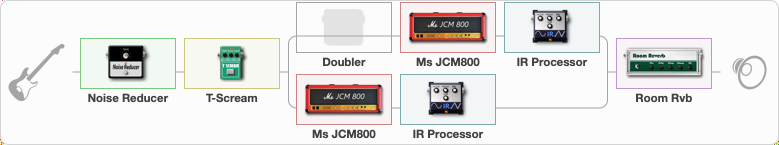# KH KILL EM ALL

Discussion in 'ToneLib-GFX presets' started by grigoriy0192873, Mar 20, 2023.

1. ### grigoriy0192873Active Member

KH KILL EM ALL

Preset name: KH KILL EM ALL

Effects chain:Effect: "Noise Reducer" (Dynamics / Filter), active - "yes"
{
"Sens" = 50
"Mode" = Hard
}

Effect: "T-Scream" (Overdrive / Distortion), active - "yes"
{
"Drive" = 0
"Tone" = 80
"Level" = 100
}

Effect: "Splitter" (Dynamics / Filter)
{
"A-Bypass" = Off
"A-Pan" = -100
"A-Level" = 50
"B-Bypass" = Off
"B-Pan" = 100
"B-Level" = 50
"Width" = 100

'A' branch:
{

Effect: "Doubler" (Dynamics / Filter), active - "no"
{
"A-Dly" = 75
"A-Inv" = Off
"A-Level" = 100
"B-Dly" = 0
"B-Inv" = Off
"B-Level" = 50
}

Effect: "Ms JCM800" (Amp simulators), active - "yes"
{
"Gain" = 80
"Bass" = 100
"Middle" = 0
"Treble" = 50
"Presence" = 0
"Master" = 40
"Level (dB)" = 12
}

Effect: "IR Processor" (Cabinets), active - "yes"
{
"IR" = killem_IR
"Low Cut (Hz)" = 0
"Hi Cut (kHz)" = 20.0
"Mix" = 100
"Level (dB)" = 0
}
}
'B' branch:
{

Effect: "Ms JCM800" (Amp simulators), active - "yes"
{
"Gain" = 80
"Bass" = 100
"Middle" = 0
"Treble" = 50
"Presence" = 0
"Master" = 40
"Level (dB)" = 11
}

Effect: "IR Processor" (Cabinets), active - "yes"
{
"IR" = killem_IR
"Low Cut (Hz)" = 0
"Hi Cut (kHz)" = 20.0
"Mix" = 100
"Level (dB)" = 0
}
}
}

Effect: "Room Rvb" (Reverberation), active - "yes"
{
"Time" = 4.5
"PreDelay" = 0
"LoDamp" = 46
"HiDamp" = 25
"Mix" = 59
}

Note: You will need to download and install the ToneLib-GFX software to use the preset.

File size:
9.2 KB
Views:
1,637